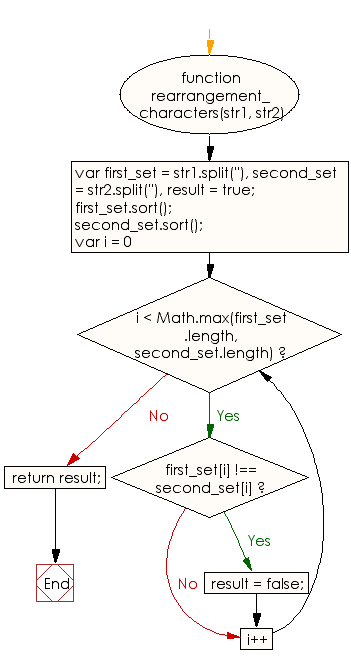# JavaScript: Rearrange characters of a given string in such way that it will become equal to another given string

## JavaScript Basic: Exercise-99 with Solution

Write a JavaScript program to check whether it is possible to rearrange characters of a given string in such way that it will become equal to another given string.

Sample Solution:

HTML Code:

``````<!DOCTYPE html>
<html>
<meta charset="utf-8">
<meta name="viewport" content="width=device-width">
<title> Rearrange characters of a given string in such way that it will become equal to another given string</title>
<body>

</body>
</html>
```
```

JavaScript Code:

``````function rearrangement_characters(str1, str2) {

var first_set = str1.split(''),
second_set = str2.split(''),
result = true;

first_set.sort();
second_set.sort();

for (var i = 0; i < Math.max(first_set.length, second_set.length); i++) {
if (first_set[i] !== second_set[i]) {
result = false;
}
}

return result;
}

console.log(rearrangement_characters("xyz", "zyx"))
console.log(rearrangement_characters("xyz", "zyp"))
```
```

Sample Output:

```true
false
```

Flowchart:ES6 Version:

``````function rearrangement_characters(str1, str2) {
const first_set = str1.split('');
const second_set = str2.split('');
let result = true;

first_set.sort();
second_set.sort();

for (let i = 0; i < Math.max(first_set.length, second_set.length); i++) {
if (first_set[i] !== second_set[i]) {
result = false;
}
}

return result;
}

console.log(rearrangement_characters("xyz", "zyx"))
console.log(rearrangement_characters("xyz", "zyp"))
``````

Live Demo:

See the Pen javascript-basic-exercise-99 by w3resource (@w3resource) on CodePen.

What is the difficulty level of this exercise?

Test your Programming skills with w3resource's quiz.

﻿

## JavaScript: Tips of the Day

Checks if a string is an anagram of another string (case-insensitive, ignores spaces, punctuation and special characters)

Example:

```const isAnagram = (str1, str2) => {
const normalize = str =>
str
.toLowerCase()
.replace(/[^a-z0-9]/gi, '')
.split('')
.sort()
.join('');
return normalize(str1) === normalize(str2);
};
console.log(isAnagram('iceman', 'cinema')); // true
```

Output:

```true
```